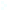Election CitationResearch Interests Name Agrawal, Manindra Location Indian Institute of Technology - Kanpur Primary Field Mathematics Secondary Field Computer and Information Sciences Gauss wrote that "The problem of distinguishing prime numbers from composite numbers is known to be one of the most important and useful in arithmetic." Agrawal and his students solved this ancient problem. He has fundamental results in Computational Number Theory and Algebra, Derandomization, Isomorphism Conjecture and Circuit Complexity. Manindra Agrawal started his research by investigating the structure of NP-complete sets, particularly the Isomorphism Conjecture which states that all NP-complete sets are isomorphic to each other under polynomial time computable isomorphisms. After a series of papers on this problem, he proved the conjecture for an interesting subclass of NP-complete problems: sets complete under reductions computable by constant depth circuits. His interest then moved on to computational number theory and algebra where he initiated a new approach for primality testing which culminated in the first deterministic polynomial time algorithm for the problem. In the last decade or so, he has worked primarily on Polynomial Identity Testing (PIT) problem: to check if a polynomial, given as a sequence of addition and multiplication operations, is non-zero. He has contributed to showing the fundamental importance of this problem to the quest of finding an explicit polynomial that requires exponential number of operations to compute. He has also been working on finding efficient algorithms for solving PIT.